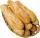# Decimal to fraction

Write decimal number 8.638333333 as a fraction A/B in the basic form. Given decimal has infinite repeating figures.

Result

A =  5183
B =  600

#### Solution:Leave us a comment of example and its solution (i.e. if it is still somewhat unclear...):Be the first to comment!#### To solve this verbal math problem are needed these knowledge from mathematics:

Need help calculate sum, simplify or multiply fractions? Try our fraction calculator.

## Next similar examples:

1. Series and sequencesFind a fraction equivalent to the recurring decimal? 0.435643564356
2. Infinite decimalImagine the infinite decimal number 0.99999999 .. ... ... ... That is a decimal and her endless serie of nines. Determine how much this number is less than the number 1. Thank you in advance.
3. SequenceFind the common ratio of the sequence -3, -1.5, -0.75, -0.375, -0.1875. Ratio write as decimal number rounded to tenth.
4. FractionFraction ? write as fraction a/b, a, b is integers numerator/denominator.
5. RollsMom bought 13 rolls. Dad ate 3.5 rolls. How many rolls left when Peter yet put two at dinner?
6. Sum of seriesDetermine the 6-th member and the sum of a geometric series: 5-4/1+16/5-64/25+256/125-1024/625+....
7. Five membersWrite first 5 members geometric sequence and determine whether it is increasing or decreasing: a1 = 3 q = -2
8. Theorem proveWe want to prove the sentence: If the natural number n is divisible by six, then n is divisible by three. From what assumption we started?
9. Imaginary numbersFind two imaginary numbers whose sum is a real number. How are the two imaginary numbers related? What is its sum?
10. A perineumA perineum string is 10% shorter than its original string. The first string is 24, what is the 9th string or term?
11. Geometric sequence 4It is given geometric sequence a3 = 7 and a12 = 3. Calculate s23 (= sum of the first 23 members of the sequence).
12. Tenth memberCalculate the tenth member of geometric sequence when given: a1=1/2 and q=2
13. GP membersThe geometric sequence has 10 members. The last two members are 2 and -1. Which member is -1/16?
14. Geometric progression 2There is geometric sequence with a1=5.7 and quotient q=-2.5. Calculate a17.
15. Six termsFind the first six terms of the sequence a1 = -3, an = 2 * an-1
16. Geometric progression 48,4√2,4,2√2
17. GP - 8 itemsDetermine the first eight members of a geometric progression if a9=512, q=2